Latest Banking jobs   »

# Quantitative Aptitude Quiz For IBPS RRB PO Prelims 2022- 20th June

Directions (1-5): The following questions are accompanied by two statements (I) and statement (II). You have to determine which statements(s) is/are sufficient/necessary to answer the questions given below.
(a) Statement (I) alone is sufficient to answer the question but statement (II) alone is not sufficient to answer the question.
(b) Statement (II) alone is sufficient to answer the question but statement (I) alone is not sufficient to answer the question.
(c) Both the statements taken together are necessary to answer the question, but neither of the statements alone is sufficient to answer the question.
(d) Either statement (I) or statement (II) by itself is sufficient to answer the question.
(e) Statements (I) and (II) taken together are not sufficient to answer the question.

Quantitative Quiz For IBPS RRB PO Prelims 2022 20th June CHECK IN HINDI

Q1. Curved surface area of a canonical box is 136π cm². Find the volume of the canonical box?
I. Slant height of the canonical Box is 17 cm.
II. Area of the base of canonical box is 64π cm².

Q2. A, B and C together can complete a piece of work in 15 days, then find in how many days B alone can complete the same piece of work?
I. Efficiency of A and C is 50% less and 25% less than the efficiency of B respectively.
II. A and B together can complete the same work in 22 ½ days and B is two times efficient than A.

Q3. Speed of train-A is 20% more than the speed of train-B and length of train-A is 120m more than length of train-B. find the Speed of train-A?
I. If train-A crosses a pole in 18 sec and train-B crosses the same pole in 72/5 sec.
II. If both trains passed each other in 180 sec while moving in opposite direction.

Q4. A shopkeeper marks price of an article 40% above its cost price and gives a discount of r% on it. Find r?
I. If cost price of the article is Rs. 2500.
II. If selling price of the article is Rs. 300 more than the cost price of the article.

Q5. A bag contains x red balls, y blue balls and 10 green balls. Find the total number of red and blue balls in the bag?
I. If one ball is drawn from bag and probability of being that ball Red is 5/22.
II. If one ball is drawn from bag and probability of being that ball Blue is 7/22.

Directions (6-10): In the following questions two quantities (Quantity I and Quantity II) are given for each question. Compare the numeric value of both the quantities and answers accordingly.

Q6. Quantity I – A boat can cover a certain distance in 50 hours and 70 hours in downstream and upstream respectively. Time taken by boat to cover same distance in still water.
Quantity II – Veer and Aayush together can complete a work in 240/7 hours and Aayush alone can complete the same work in 80 hours. Time taken by Veer to complete same work alone.
(a) Quantity I > Quantity II
(b) Quantity I < Quantity II
(c) Quantity I ≥ Quantity II
(d) Quantity I ≤ Quantity II
(e) Quantity I = Quantity II or no relation

Q7. Quantity I – Find ‘x’, if x+1/x = 2
Quantity II– Find radius of circle. Area of a circle is 35% of its circumference of that Circle. (consider only numeric values)
(a) Quantity I > Quantity II
(b) Quantity I < Quantity II
(c) Quantity I ≥ Quantity II
(d) Quantity I ≤ Quantity II
(e) Quantity I = Quantity II or no relation

Q8. If present age of Shiva is 16⅔% less than the present age of Rajeev. Seven years ago, the Rajeev’s age was 25% more than Shiva’s age at that time.
Quantity I: Present age of Shiva
Quantity II: Seven years ago, Rajeev Age.
(a) Quantity I > Quantity II
(b) Quantity I < Quantity II
(c) Quantity I = Quantity II or No relation
(d) Quantity I ≥ Quantity II
(e) Quantity I ≤ Quantity II

Q9. The Perimeter of an isosceles right-angle triangle is (16+8√2) cm.
Quantity I : Find out the radius of circle which is inscribed in the triangle.
Quantity II : Find out the value of 75% of the radius of circumcircle of the triangle.
(a) Quantity I > Quantity II
(b) Quantity II > Quantity I
(c) Quantity I ≥ Quantity II
(d) Quantity II ≥ Quantity I
(e) Quantity I = Quantity II or relation can’t be established

Q10. A container contains 60 liters mixture of alcohol and water.
Quantity I. Initial quantity of alcohol. if 6 liters water is added in the container then ratio of alcohol to water becomes 6:5.
Quantity II: 60% more than initial quantity of water. if 15 liters alcohol is added in the container then ratio of alcohol to water becomes 8:7.
(a) Quantity I > Quantity II
(b) Quantity I < Quantity II
(c) Quantity I ≥ Quantity II
(d) Quantity I ≤ Quantity II
(e) Quantity I = Quantity II or no relation

Directions (11-15) In each of the questions below consists of a question and two statements numbered I and II given below it. You have to decide whether the data provided in the statements are sufficient to answer the question. Read both the statements and give answers accordingly.

(a) If the data in statement I alone sufficient to answer the question, while the data in statement II alone not sufficient to answer the question
(b) If the data in statement II alone sufficient to answer the question, while the data in statement I alone not sufficient to answer the question
(c) If the data either in statement I alone or in statement II alone sufficient to answer the question
(d) If the data given in both statements I and II together are not sufficient to answer the question
(e) If the data in both statements I and II together are necessary to answer the question.

Q11: What is present age of Shubham?
Statement I. Shubham’s present age is 33⅓% of his mother’s present age.
Statement II. Shubham’s brother present age is 11-year and he is 34 years younger to his mother.

Q12. In how many days A, B and C together can complete the work?
Statement I: A alone can complete half of the work in 7 days.
Statement II: Ratio of efficiency B and C is 2:3 respectively.

Q13. Is y greater than z?
Statement I: 1/y is less than z.
Statement II: 1/z is less than 1/y.

Q14. How much time train R will take to cover a distance of 450 km?
Statement I: The ratio of speed of train R and train Q is 4 : 5 respectively.
Statement II: The average of speed of the train R and train Q is 27/2 km/hour.

Q15. What is the cost price of bike?
Statement I: A shopkeeper marks up bike 60% above the cost price and allows a discount of 25% on it and thus makes a profit of Rs. 24000.
Statement II: A shopkeeper sold the bike at Rs. 150000 and makes a profit of 25%.

Solutions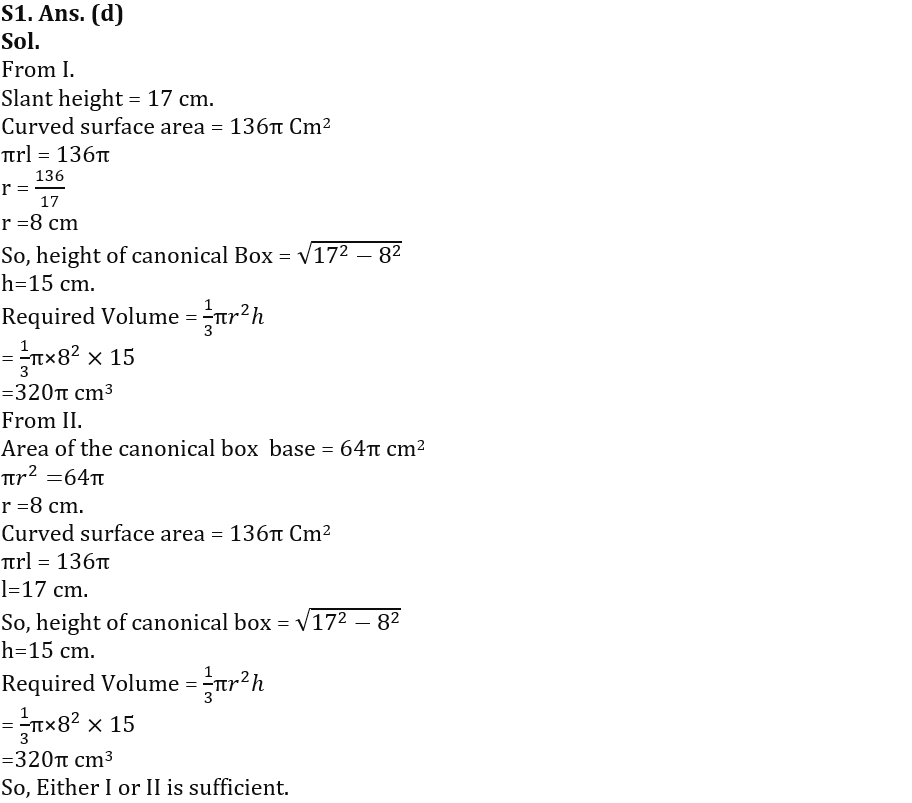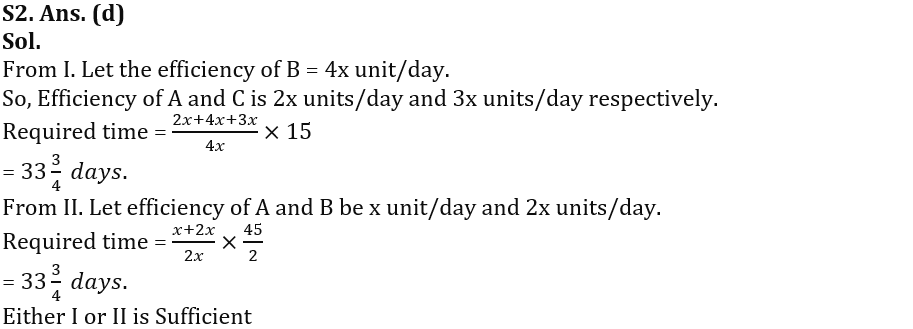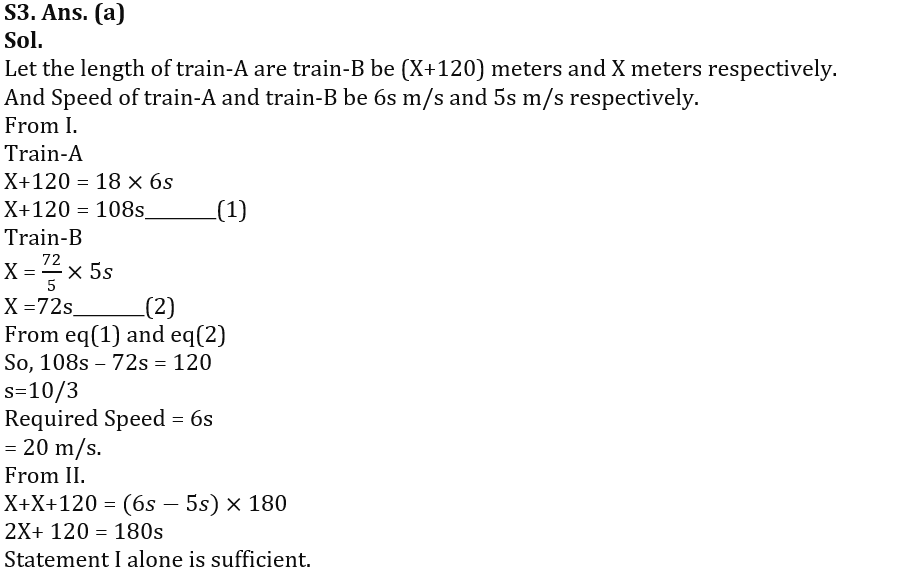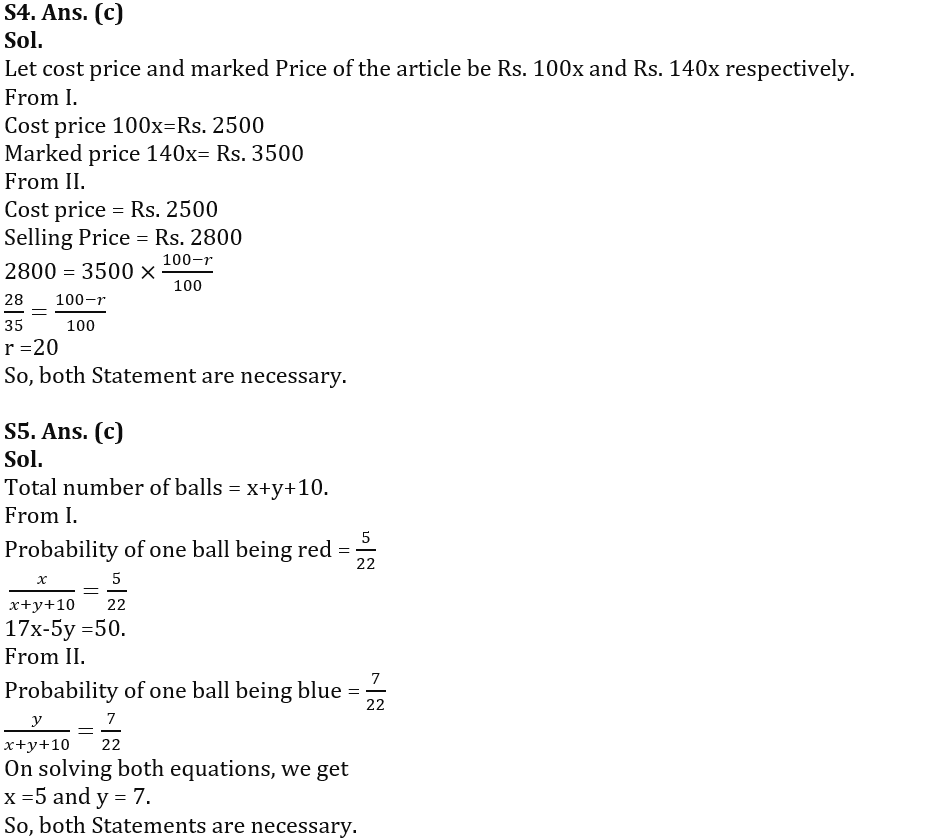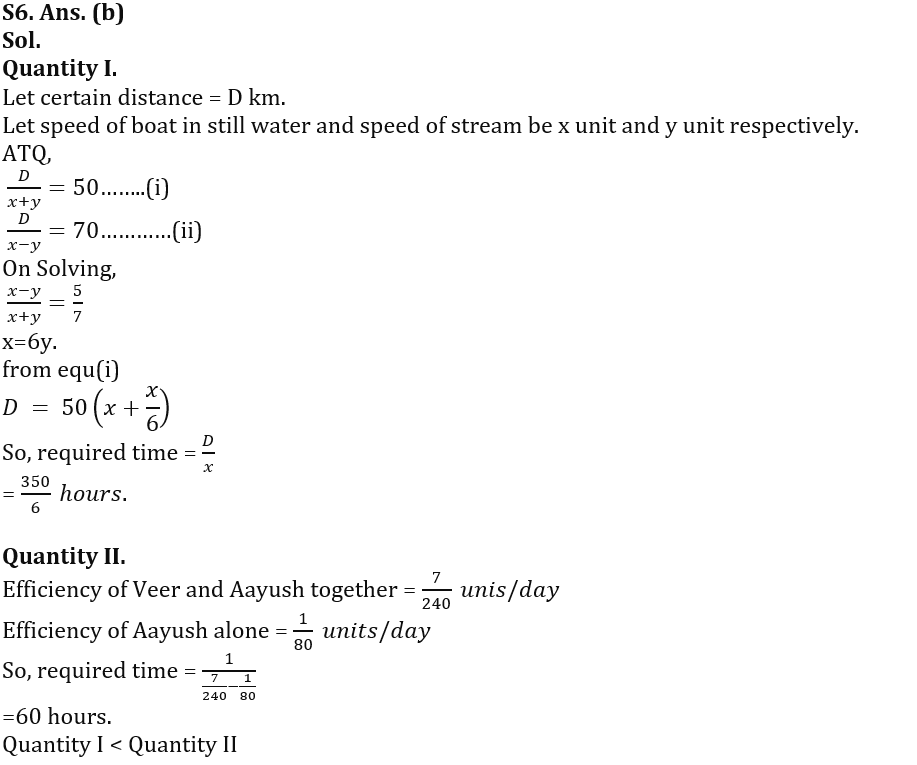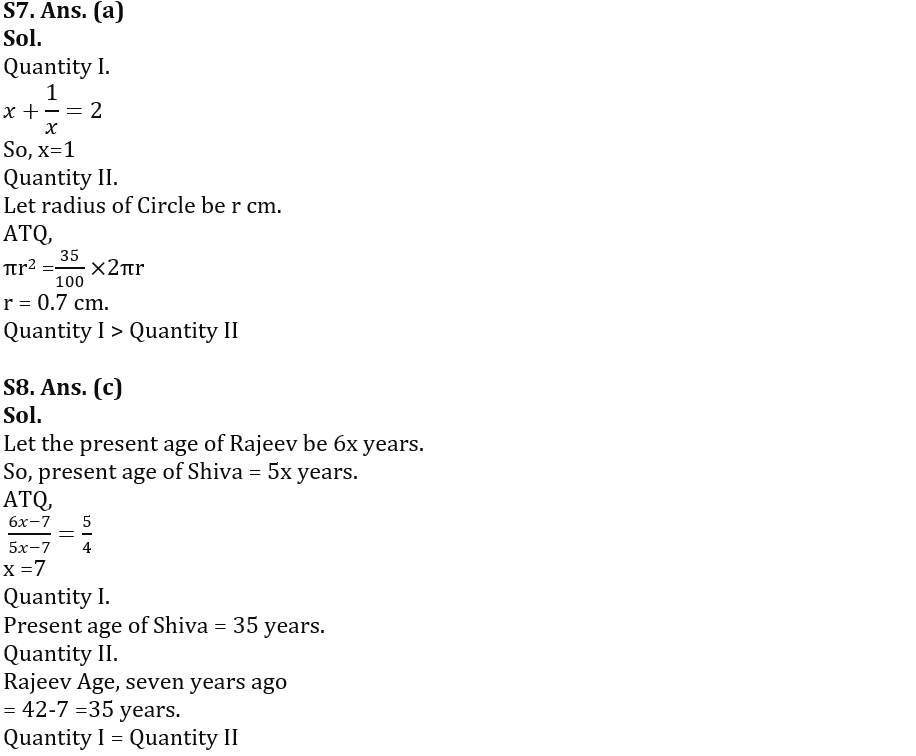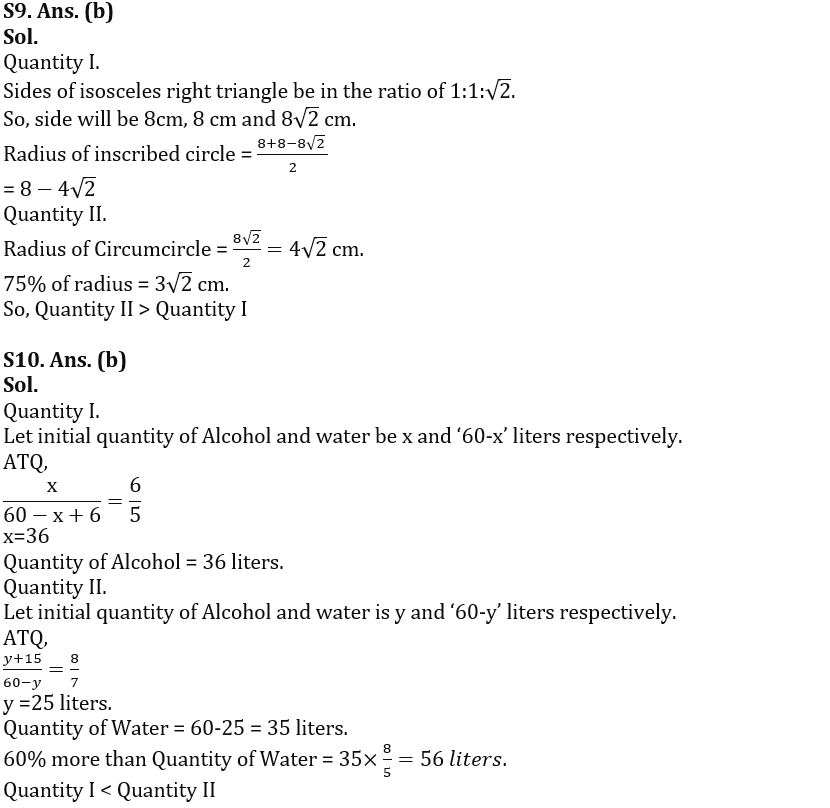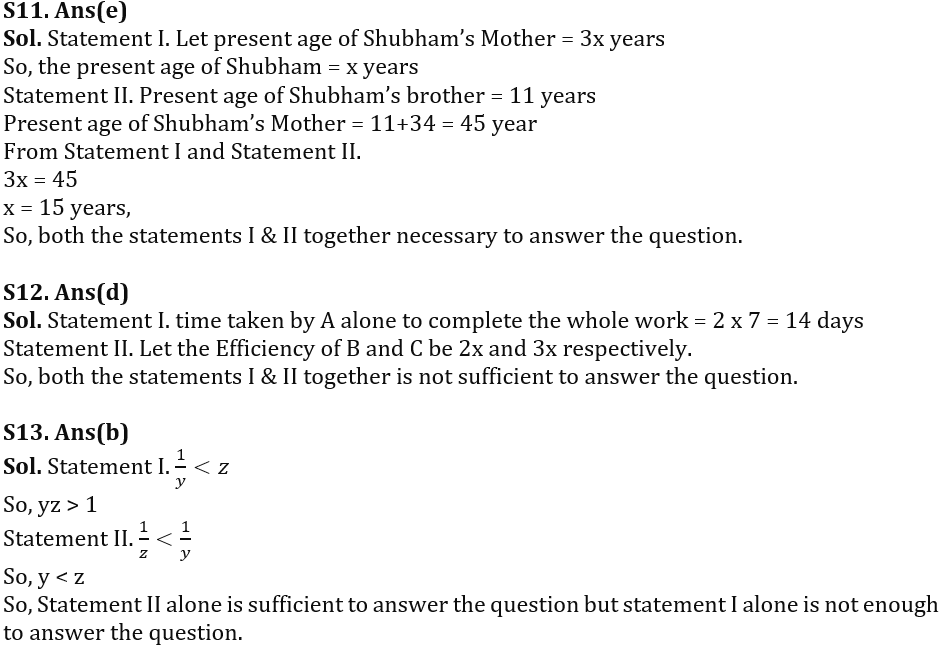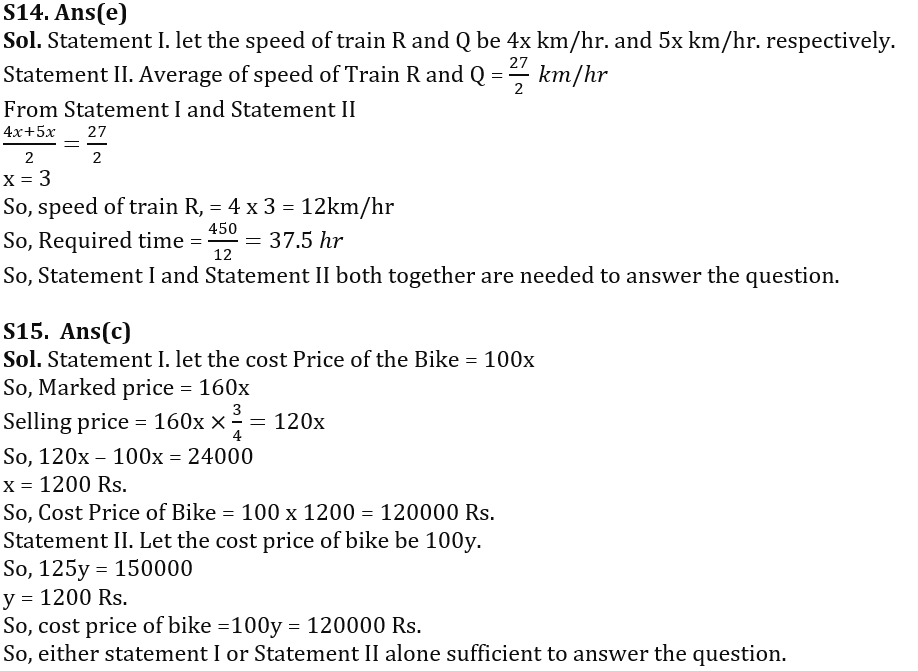#### Congratulations!